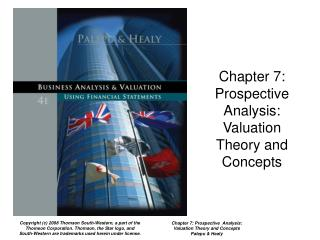DownloadDownload PresentationChapter 7: Prospective Analysis: Valuation Theory and Concepts

# Chapter 7: Prospective Analysis: Valuation Theory and Concepts

Télécharger la présentation## Chapter 7: Prospective Analysis: Valuation Theory and Concepts

- - - - - - - - - - - - - - - - - - - - - - - - - - - E N D - - - - - - - - - - - - - - - - - - - - - - - - - - -
##### Presentation Transcript

1. Chapter 7: Prospective Analysis: Valuation Theory and Concepts Chapter 7: Prospective Analysis: Valuation Theory and Concepts Palepu & Healy

2. Key Concepts in Chapter 7 • Forecasts (Ch. 6) are converted into estimates of value. • Discounted future dividends, cash flows, and abnormal earnings may be used to estimate value. • Price-based multiples may also be used as value estimates. • No method by itself dominates any of the others. Chapter 7: Prospective Analysis: Valuation Theory and Concepts Palepu & Healy

3. Discounted Dividends Valuation • The present value of future cash flows to shareholders is the basis of the discounted dividends method. • This method is the basis for most theoretical approaches to stock valuation, including the other methods discussed in this chapter. Where re is the cost of equity capital Chapter 7: Prospective Analysis: Valuation Theory and Concepts Palepu & Healy

4. Discounted Abnormal Earnings • Abnormal earnings are those that differ from the expected return: NIt – re * BVE0 • The discounted dividends method can be modified to yield the following relationship: • Equity value = BVE0 + PV expected future abnormal earnings Chapter 7: Prospective Analysis: Valuation Theory and Concepts Palepu & Healy

5. Accounting Methods and Discounted Abnormal Earnings • Analysts must recognize the impact of different accounting methods on value estimates • Valuations are based on earnings and book values • Accounting choices affect earnings and book values • Double-entry bookkeeping is by nature self-correcting • Strategic and accounting analyses are important steps to precede abnormal earnings valuation. Chapter 7: Prospective Analysis: Valuation Theory and Concepts Palepu & Healy

6. Price Multiples Valuation • Price multiple valuation methods are popular because of their simplicity. • Three steps are involved: 1. Select base measure 2. Calculate price multiples for comparable firms 3. Apply comparable firm multiple to firm analyzed Chapter 7: Prospective Analysis: Valuation Theory and Concepts Palepu & Healy

7. Precautions in Using Price Multiples Valuation • Selecting comparable firms • It may be difficult to identify comparable firms, even within an industry • Industry averages may be used instead • Firms with poor performance • Marginal profitability or earnings shocks must be considered • Adjustments for leverage • Take care to maintain consistency between numerator and denominator Chapter 7: Prospective Analysis: Valuation Theory and Concepts Palepu & Healy

8. Determinants of Value to Book/Earnings Multiples • Value-to-book ratio is driven largely by: • Magnitude of future abnormal ROEs • Growth in book value • Equity value-earnings can be derived from the value-to-book formula: Chapter 7: Prospective Analysis: Valuation Theory and Concepts Palepu & Healy

9. ROE, Equity Growth, Price-to-Book Ratio, and Price-Earnings Ratio Chapter 7: Prospective Analysis: Valuation Theory and Concepts Palepu & Healy

10. Shortcut Forms of Earnings-Based Valuation • Assumptions may be made to simplify abnormal earnings and equity value-to-book methods. • Abnormal earnings: random walk and autoregressive models • ROE and Growth: ROE mean reversion, other assumptions (e.g., decay) Chapter 7: Prospective Analysis: Valuation Theory and Concepts Palepu & Healy

11. Discounted Cash Flow Model • Derived from the discounted dividends model Equity value = PV free cash flows to equity claim holders Requires: 1. Forecasts of fee cash flows (usually 5 – 10 years) 2. Forecasts of fee cash flows beyond terminal year 3. Discounting free cash flows using the cost of equity Chapter 7: Prospective Analysis: Valuation Theory and Concepts Palepu & Healy

12. Comparing Valuation Methods • No one method is superior to the others • Using the same assumptions about firm fundamentals should yield the same value estimates from either of the three methods used. • The three methods differ in the following aspects: Focus – earnings or cash flow Amount of analysis or structure required Terminal value implications Chapter 7: Prospective Analysis: Valuation Theory and Concepts Palepu & Healy

13. Concluding Comments • The value of a stock is the present value of future dividends. • Three methods are derived from this rule: • Discounted dividends • Abnormal earnings • Discounted cash flows • Each of these methods focuses the analyst’s attention on different issues and requires a different level of structure to develop forecasts of the underlying dividends Chapter 7: Prospective Analysis: Valuation Theory and Concepts Palepu & Healy